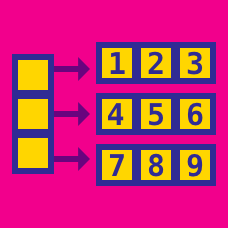Computer Science

# Linear Data Structures: Level 4 Challenges

Given an array of unique randomly sorted integers and a number $k$ it is required to find the count of the unordered pairs in the array that have a difference of $k$. In the text file, how many unorederd pairs of numbers have a difference of 70.

Details and Assumptions

As an example in the array [2, 8, 4, 3, 1] with $k=2$ is $2$....(2,4) and (3,1).The various stock prices of an item during various points throughout the day are given in this text. What is the maximum profit you can get if you are allowed to perform at most two buys and at most two sells?

Details and Assumptions

• You can only buy another stock if you have sold the one you bought previously.

• As an explicit example if the stock prices are [2, 4, 5, 6, 9, 5, 4, 3, 8] you buy at $2$ and sell at $9$, and you buy another stock at $3$ and sell at $8$. for a max profit of $12$

• [8, 7, 5, 4, 3, 2] there will be no profit, as the value of the stock is decreasing with time.

• The stock prices are sequential with respect to time, so that you can only buy or sell only the stock at hand.

##### Previous programming contest problemThere is an array $A_{1}$ and another array $A_{2}$ which is an exact copy of the first array. By accident one of the numbers from the second array is deleted. What is the deleted number?

Note: array1 and array2.

×